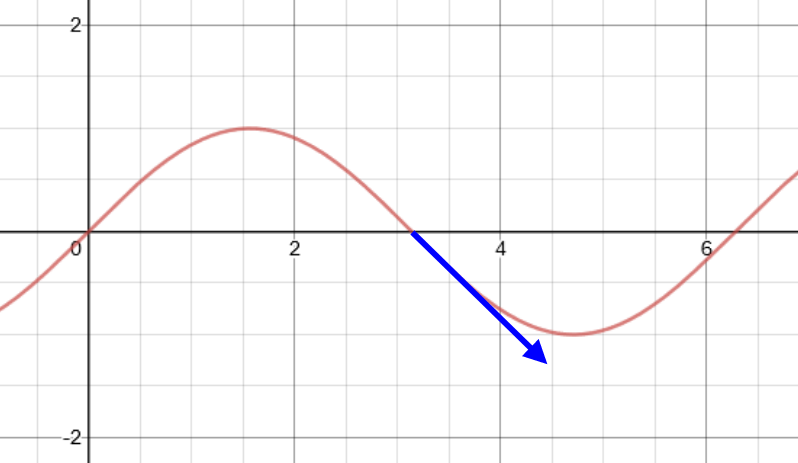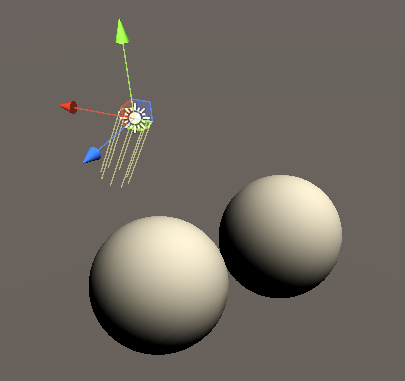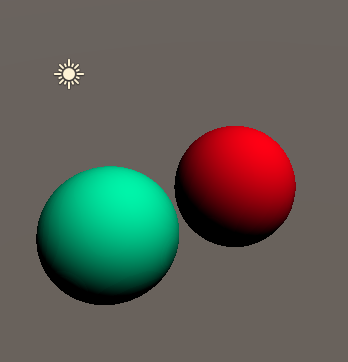In a previous tutorial I showed how to trace signed distance functions to reveal their silouette. In this one I will show you how to expand that shader to add simple lighting and make the objects look more tangible.

## Architecture Changes

In the previous shader we returned a solid color after finding a surface the ray collides with. To add lighting or other effects we have to expand this part. To keep the shader as readable as possible we’ll do a function call in this place and return the result of the function. This material function will calculate the light and combine the lighting with the surface color. To make this function as modular as possible I decided to put the light calculations in another function and the calculations for the normal of the surface in yet another one. If fewer more monolithic functions are more readable to you, feel free to structure your shader like that.

``````float3 normal(float3 pos){
//calculate surface normal
}

float3 lightColor(float3 pos){
//calculate light color
}

float4 material(float3 pos){
//return final surface color
}

fixed4 frag(v2f i) : SV_TARGET{
//ray information
float3 pos = i.localPosition;
float3 dir = normalize(i.viewDirection.xyz);
float progress = 0;

//tracing loop
for (uint iter = 0; iter < MAX_STEPS; iter++) {
//get current location on ray
float3 samplePoint = pos + dir * progress;
//get distance to closest shape
float distance = scene(samplePoint);
//return color if inside shape
if(distance < THICKNESS){
return material(samplePoint);
}
//go forwards
progress = progress + distance;
}
//discard pixel if no shape was hit
clip(-1);
return 0;
}
``````

## Normal

One of the most important variables when calculating light in a shader is the surface normal. Unlike a mesh where the normal is embeded into the data, we have to get the normal from the scene function ourselves in this case. We use the fact that we can see a signed distance field as a function to calculate the normal. The normal is the direction in which the value of the SDF grows. Even though a 3d function looks way more complex than a 1d function we can still use similar techniques to find the direction of it.

This “direction” of a function is also called its “derivative” and a common and simple way to find it is to look at two close points of the function and compare the change in value. With 1d functions we pass the function 2 different X values and divide the change in resulting Y values by the change in the X axis to get the rate at which the result of the function changes.When working with our 3d signed distance function we can do the same separately in 3 axis and since we only care about the direction and use the same change in position in all axis we don’t even have to care about the length of the resulting vector.

We call the change in position to get the normal “epsilon”. A epsilon that’s too big leads to surfaces that look too smooth and inaccurate, but a epsilon that’s too small can lead to calculation imprecisions, so it’s worth playing around with that value for your use case. I chose 0.01 as a starting value. The function to calculate the change in signed distance value is scenevalue at the position plus a little distance in a axis subtracted by the scene value at the position minus a tiny number on that axis. In code this looks like this:

``````//determine change in signed distance
float changeX = scene(pos + float3(NORMAL_EPSILON, 0, 0)) - scene(pos - float3(NORMAL_EPSILON, 0, 0));
float changeY = scene(pos + float3(0, NORMAL_EPSILON, 0)) - scene(pos - float3(0, NORMAL_EPSILON, 0));
float changeZ = scene(pos + float3(0, 0, NORMAL_EPSILON)) - scene(pos - float3(0, 0, NORMAL_EPSILON));
``````

You can see how to get the derivative on the X axis we move the position a bit to the right and left on that axis.

After getting all those values we can combine them to one normal vector. Since we used such a tiny epsilon, the normal vector will also be very small, so to fix that we normalize it wich results in a normal vector with a length of 1. Since we decided the object is traced in object space, but a normal in worldspace is more useful we also do a matrix multiplication to convert it into worldspace before normalizing it. For the multiplication we add a 4th component which we set to 0, this makes the instruction only change the rotation and scale of the vector, but doesn’t move it since the normal is position independent.

``````//distance from rendered point to sample SDF for normal calculation
#define NORMAL_EPSILON 0.01

float3 normal(float3 pos){
//determine change in signed distance
float changeX = scene(pos + float3(NORMAL_EPSILON, 0, 0)) - scene(pos - float3(NORMAL_EPSILON, 0, 0));
float changeY = scene(pos + float3(0, NORMAL_EPSILON, 0)) - scene(pos - float3(0, NORMAL_EPSILON, 0));
float changeZ = scene(pos + float3(0, 0, NORMAL_EPSILON)) - scene(pos - float3(0, 0, NORMAL_EPSILON));
//construct normal vector
float3 surfaceNormal = float3(changeX, changeY, changeZ);
//convert normal vector into worldspace and make it uniform length
surfaceNormal = mul(unity_ObjectToWorld, float4(surfaceNormal, 0));
return normalize(surfaceNormal);
}
``````

If we now return the result of the normal function from the shader, we can see the worldspace normals which lead to a red/green/blue surface in the same direction as the direction gizmo in the corner.

``````//return color if inside shape
if(distance < THICKNESS){
return float4(normal(samplePoint), 1);
}
``````## Lighting

The lighting function works the same as in any other context. The main difference to the lighting implementations I’ve explained previously we can’t use surface shaders so this implementation is more limited since that would need multiple shader passes. I’m not going to implement more than 1 light and won’t show how to make point lights work here, so we’re stuck with a single directional light.

We begin my retrieving the surface normal with the previously written function. Then we get the direction the light is coming from. In the case of directional lights this is always saved in `_WorldSpaceLightPos0.xyz`.

With this information we can do a simple lighting calculation. We get the dot product between direction and normal and then we use the saturate function to ensure the result is never negative. The result of the function is this falloff multiplied by the color of the light which is stored in `_LightColor0` to use the color of the light as a tint. It’s important that the `_LightColor0` variable is only available when we imclude the `Lighting.cginc` include file in our shader, so we also add that.

``````#include "Lighting.cginc"

float4 lightColor(float3 position){
//calculate needed surface and light data
float3 surfaceNormal = normal(position);
float3 lightDirection = _WorldSpaceLightPos0.xyz;

float lightAngle = saturate(dot(surfaceNormal, lightDirection));
return lightAngle * _LightColor0;
}
``````

Printing out the result of the lighting function already looks like a plain white surface.

``````//return color if inside shape
if(distance < THICKNESS){
return lightColor(samplePoint);
}
``````## Final Steps

The last step for this shader I want to show is how to include the surface color into the shader again. For this we prepared the material function earlier which combines everything into the final result. In this implementation we just multiply the light we calculated with the color property which we added in the previous tutorial to get the final color.

``````float4 material(float3 position){
//get light color
float4 light = lightColor(position);

//combine base color and light color
float4 color = _Color * light;

return color;
}
``````You can expand this by reading a texture or generating a pattern in the material function or by using more complex or interresting lighting functions in the lightColor function and of course by using a more complex signed distance field, but I hope this tutorial gave you some insight into the basics and how to get to more complex implementations.

## Source

``````Shader "Tutorial/044_SphereTracingShading"{
//show values to edit in inspector
Properties{
_Color ("Color", Color) = (0, 0, 0, 1)
}

//the material is completely non-transparent and is rendered at the same time as the other opaque geometry
Tags{ "RenderType"="Opaque" "Queue"="Geometry" "DisableBatching"="True"}

Pass{
ZWrite Off

CGPROGRAM
#include "UnityCG.cginc"
#include "Lighting.cginc"

#pragma vertex vert
#pragma fragment frag

//surface color
fixed4 _Color;

//maximum amount of steps
#define MAX_STEPS 10
//furthest distance that's accepted as inside surface
#define THICKNESS 0.01
//distance from rendered point to sample SDF for normal calculation
#define NORMAL_EPSILON 0.01

//input data
struct appdata{
float4 vertex : POSITION;
};

//data that goes from vertex to fragment shader
struct v2f{
float4 position : SV_POSITION; //position in clip space
float4 localPosition : TEXCOORD0; //position in local space
float4 viewDirection : TEXCOORD1; //view direction in local space (not normalized!)
};

v2f vert(appdata v){
v2f o;
//position for rendering
o.position = UnityObjectToClipPos(v.vertex);
//save local position for origin
o.localPosition = v.vertex;
//get camera position in local space
float4 objectSpaceCameraPos = mul(unity_WorldToObject, float4(_WorldSpaceCameraPos, 1));
//get local view vector
o.viewDirection = v.vertex - objectSpaceCameraPos;
return o;
}

float scene(float3 pos){
return length(pos) - 0.5;
}

float3 normal(float3 pos){
//determine change in signed distance
float changeX = scene(pos + float3(NORMAL_EPSILON, 0, 0)) - scene(pos - float3(NORMAL_EPSILON, 0, 0));
float changeY = scene(pos + float3(0, NORMAL_EPSILON, 0)) - scene(pos - float3(0, NORMAL_EPSILON, 0));
float changeZ = scene(pos + float3(0, 0, NORMAL_EPSILON)) - scene(pos - float3(0, 0, NORMAL_EPSILON));
//construct normal vector
float3 surfaceNormal = float3(changeX, changeY, changeZ);
//convert normal vector into worldspace and make it uniform length
surfaceNormal = mul(unity_ObjectToWorld, float4(surfaceNormal, 0));
return normalize(surfaceNormal);
}

float4 lightColor(float3 position){
//calculate needed surface and light data
float3 surfaceNormal = normal(position);
float3 lightDirection = _WorldSpaceLightPos0.xyz;

float lightAngle = saturate(dot(surfaceNormal, lightDirection));
return lightAngle * _LightColor0;
}

float4 material(float3 position){
//get light color
float4 light = lightColor(position);

//combine base color and light color
float4 color = _Color * light;

return color;
}

fixed4 frag(v2f i) : SV_TARGET{
//ray information
float3 pos = i.localPosition;
float3 dir = normalize(i.viewDirection.xyz);
float progress = 0;

//tracing loop
for (uint iter = 0; iter < MAX_STEPS; iter++) {
//get current location on ray
float3 samplePoint = pos + dir * progress;
//get distance to closest shape
float distance = scene(samplePoint);
//return color if inside shape
if(distance < THICKNESS){
return material(samplePoint);
}
//go forwards
progress = progress + distance;
}
//discard pixel if no shape was hit
clip(-1);
return 0;
}

ENDCG
}
}
}
``````

You can also find me on twitter at @totallyRonja. If you liked my tutorial and want to support me you can do that on Patreon (patreon.com/RonjaTutorials) or Ko-Fi (ko-fi.com/RonjaTutorials).